#### Particle filtering

As mentioned so far, the discrete distributions can be estimated by using samples. In fact, it turns out that the Voronoi regions over the samples do not even need to be carefully considered. One can work directly with a collection of samples drawn randomly from the initial probability density,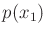. The general method is referred to as particle filtering and has yielded good performance in applications to experimental mobile robotics. Recall Figure 1.7 and see Section 12.2.3.

Let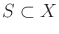denote a finite collection of samples. A probability distribution is defined over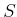. The collection of samples, together with its probability distribution, is considered as an approximation of a probability density over. Sinceis used to represent probabilistic I-states, let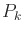denote the probability distribution over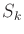, which is computed at stageusing the history I-state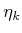. Thus, at every stage, there is a new sample set,, and probability distribution,.

The general method to compute the probabilistic I-state update proceeds as follows. For some large number,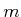, of iterations, perform the following:

1. Select a state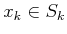according to the distribution.
2. Generate a new sample,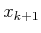, for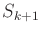by generating a single sample according to the density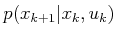.
3. Assign the weight,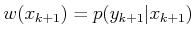.
After theiterations have completed, the weights overare normalized to obtain a valid probability distribution,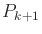. It turns out that this method provides an approximation that converges to the true probabilistic I-states astends to infinity. Other methods exist, which provide faster convergence . One of the main difficulties with using particle filtering is that for some problems it is difficult to ensure that a sufficient concentration of samples exists in the places where they are needed the most. This is a general issue that plagues many sampling-based algorithms, including the motion planning algorithms of Chapter 5.

Steven M LaValle 2020-08-14